# A map 4

A map represents every 4 miles with 1 inch. If a school and a bank are actually 12 miles apart, how far apart are they on the map, in inches?

m =  3 in

### Step-by-step explanation: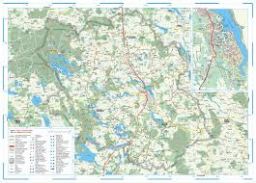Did you find an error or inaccuracy? Feel free to write us. Thank you!Tips to related online calculators
Do you want to convert length units?

## Related math problems and questions:

• Two citiesOn a map, 1.5 cm represents 50 miles. If the distance between the two cities on the map is 6 cm, how many miles separate the cities?
• Map scale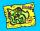What is the scale of the map, if 5.4 km is 72 mm on the map?
• The scaleThe distance of 15 cm on the map corresponds to a distance of 27 km in reality. What is the scale of the map?
• Scale factor 2Nadeem has a 10 inch by 10 inch photo album. He wants to make as large a copy of the original photo as possible to fit the album. Whats are the largest dimensions the copy can be if he keeps the proportion of the original photo? Explain your answer. .. .
• Scale of the map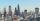The distance between two cities is actually 30 km and the map is 6 cm. What is the scale of the map?
• A map 3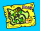A map is drawn to a scale of 1 : 25 000. This scale can be expressed as 1 cm represents n km. Find n.
• Nautical miles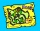How many nautical miles do they sail if the route is shown on a 1:25 000 scale map with a 7.4 cm long line?
• Map scaleGarden has on plan on a scale of 1: 150 width 22 cm and length 35 cm. What is the real area of the garden?
• On the mapA line 1.5 cm long corresponds to a line 3 cm long on the map. What is the scale of the map?
• Map 2At what scale is made map if the distance 8.2 km corresponds on the map segment 5 cm long?
• Two monuments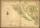The distance between two historical monuments on a map with a scale of 1: 500000 is 48 mm. Find the actual distance between these monuments in km.
• Small city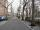The length of the street is 230 m. What will be the length of its image on the plan at a scale of 1: 2500?
• Central Park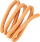Central Park is a rectangular park in New York City and the map scale is 1 cm: 320 m (It means 1 cm on the map represents 320 m in the real world. ). Find the north-south distance if the length on the map is 2.5 cm.
• Scale of the mapDetermine the map's scale, which is the actual distance of 120 km l represented by a segment long 6 cm.
• The sidewalk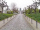The sidewalk is 50 m long. What will be its length on the plot plan on a scale of 1: 200?
• Scale of the mapDetermine the scale of the map if the actual distance between A and B is 720 km and the distance on the map is 20 cm.
• Two villages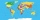Two villages are 11 km and 500 m away. On the map, their distance is determined by a 5 cm long line. Find the scale of the map.